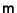## Determinant Theorem

Given a Matrix, the following are equivalent:

1..

2. The columns of m are linearly independent.

3. The rows of m are linearly independent.

4. Range(m) =.

5. Null(m) =.

6. m has a Matrix Inverse.